M408M Learning Module Pages
Main page

### Chapter 12: Vectors and the Geometry of Space

#### Learning module LM 12.6: Surfaces:

Surfaces and traces
Level curves
Level surfaces
Worked problems

# Level surfaces

Level Surfaces
Now let's step up a dimension and consider functions $w=f(x,\,y,\,z) : U \subseteq {\mathbb R}^3 \to {\mathbb R}$ of $3$ variables; one such function is $${\color{darkerblue} w \ = \ f(x,\,y,\, z) \ = \ x^2 + y^2 - z^2\,.}$$ The graph of every function $w = f(x,\,y,\, z)$ will be a surface in ${\mathbb R}^4$, though it can't be drawn directly; however, slicing horizontally by $w = c$ produces relations $c = f(x,\,y,\, z)$ in $x,\, y,$ and $z$ whose graphs will be surfaces in $3$-space which can be drawn. Formally,

 Level surfaces: For a function $w=f(x,\,y,\,z) :\, U \,\subseteq\, {\mathbb R}^3 \to {\mathbb R}$ the level surface of value $c$ is the surface $S$ in $U \subseteq {\mathbb R}^3$ on which $f\Bigl|_{S} = c\,$.

Example 1: The graph of $z=f(x,\,y)$ as a surface in $3$-space can be regarded as the level surface $w = 0$ of the function $w(x,\,y,\,z) = z - f(x,\, y)$.

Example 2: Spheres $x^2+y^2+z^2 = r^2$ can be interpreted as level surfaces $w = r^2$ of the function $w = x^2+y^2+z^2$. Can you see how to interpret ellipsoids in the same way?

From the earlier example of $w = f(x,\,y,\, z) = x^2 + y^2 - z^2$. we obtain three particularly important surfaces as level surfaces: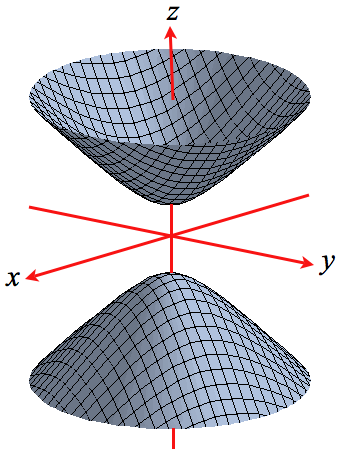$$x^2+y^2-z^2 \,=\,-1$$ Two-sheeted Hyperboloid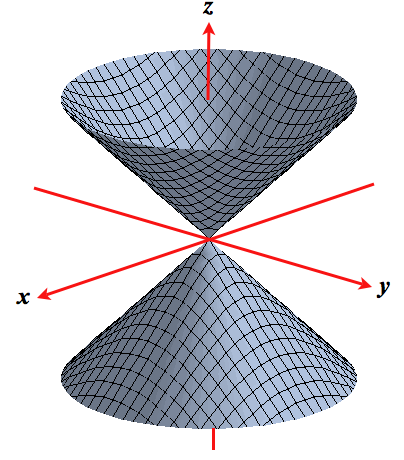$$x^2+y^2 - z^2 \,=\, 0$$ Double Cone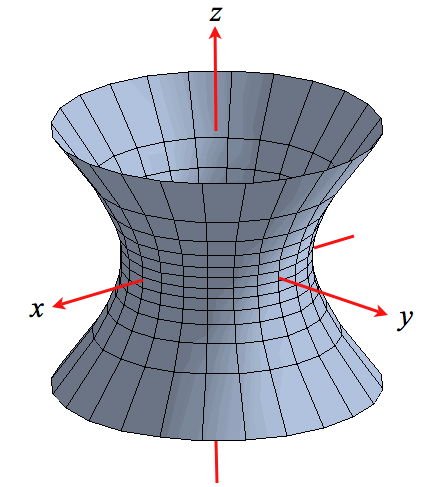$$x^2+y^2 - z^2 \,=\, 1$$ Single-sheeted Hyperboloid

by taking $c = -1,\ 0,$ and $1$. The two-sheeted hyperboloid and double cone are very important in physics, while the single sheeted hyperboloid is a favorite architectural device - cooling towers etc - as is the hyperbolic paraboloid.
Again we can investigate what happens as these surfaces are sliced by planes parallel to the coordinate planes:

Recognize the curves of intersection? There must be some underlying mathematical theory! To do no more than hint at what that theory might be notice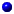all the surfaces have been the graph of some quadratic relation in $x,\, y,$ and $z$ like $z - x^2 + y^2 = 0$ in the case of a hyperbolic paraboloid or $x^2 + y^2 + z^2 = r^2$ for a sphere,all the cross-sections of these surfaces have been conic sections like parabolas, hyperbolas etc.

In view of the first of these comments we make the following

 Definition: a surface $S$ in $3$-space is said to a Quadric Surface when it is the graph of a quadratic relation in $x,\, y,\,$ and $z$. In particular, all the surfaces described so far are Quadric Surfaces.

Cylindrical Surfaces: sometimes the intersection of a surface in $3$-space with horizontal planes $z = c$ is the same for all $c$ as in the surface below to the left, or is the same for all vertical planes, say $x = a$, as in the surface to the right.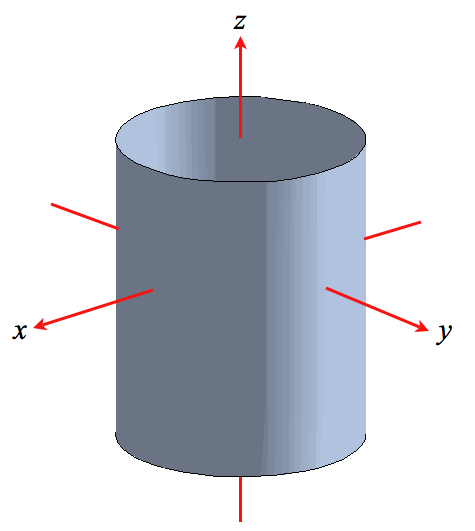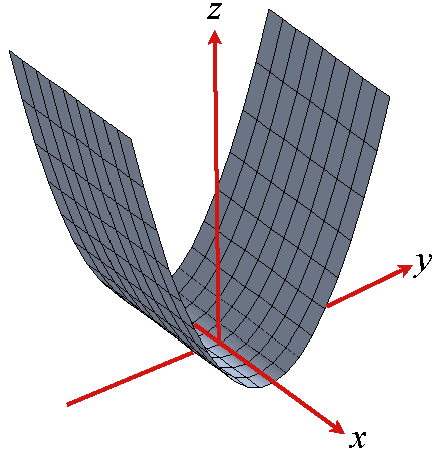Do you see that the circular cylinder to the left is the graph in $3$-space of $x^2+ y^2 = r^2$ for fixed $r$ because every horizontal slice is the same circle of radius $r$? Similarly, the cylinder to the right is parabolic; it's the graph of, say, $z = y^2$, since the intersection with every vertical plane $x = a$ is the same parabola $z = y^2$, say. Not surprisingly, it's called a Parabolic cylinder.